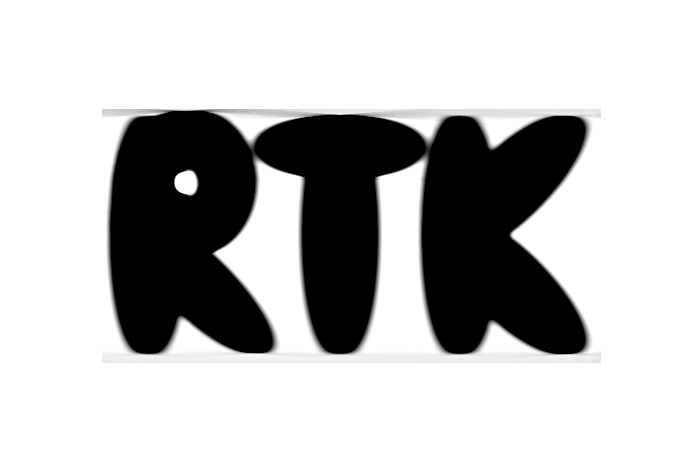RTK  2.1.0 Reconstruction Toolkit
rtk::DownsampleImageFilter< TInputImage, TOutputImage > Class Template Reference

#include <rtkDownsampleImageFilter.h>Inheritance diagram for rtk::DownsampleImageFilter< TInputImage, TOutputImage >:Collaboration diagram for rtk::DownsampleImageFilter< TInputImage, TOutputImage >:

## Public Types

using ConstPointer = itk::SmartPointer< const Self >

using InputImageConstPointer = typename InputImageType::ConstPointer

using InputImagePointer = typename InputImageType::Pointer

using InputImageType = TInputImage

using OutputImagePointer = typename OutputImageType::Pointer

using OutputImageRegionType = typename TOutputImage::RegionType

using OutputImageType = TOutputImage

using Pointer = itk::SmartPointer< Self >

using Self = DownsampleImageFilter

using Superclass = itk::ImageToImageFilter< TInputImage, TOutputImage >

## Public Member Functions

virtual ::itk::LightObject::Pointer CreateAnother () const

void GenerateInputRequestedRegion () override

void GenerateOutputInformation () override

virtual const char * GetNameOfClass () const

void SetFactor (unsigned int dimension, unsigned int factor)

void SetFactors (const unsigned int factors[])

## Static Public Member Functions

static Pointer New ()

## Static Public Attributes

static constexpr unsigned int ImageDimension = TInputImage::ImageDimension

## Protected Member Functions

DownsampleImageFilter ()

~DownsampleImageFilter () override=default

## Private Attributes

unsigned int m_Factors [ImageDimension]

int m_Offsets [ImageDimension]

## Detailed Description

### template<class TInputImage, class TOutputImage = TInputImage> class rtk::DownsampleImageFilter< TInputImage, TOutputImage >

Downsamples an image by a factor in each dimension.

This filter is inspired from Dan Mueller's GIFT package http://www.insight-journal.org/browse/publication/103

Definition at line 38 of file rtkDownsampleImageFilter.h.

## ◆ ConstPointer

template<class TInputImage , class TOutputImage = TInputImage>
 using rtk::DownsampleImageFilter< TInputImage, TOutputImage >::ConstPointer = itk::SmartPointer

Definition at line 47 of file rtkDownsampleImageFilter.h.

## ◆ InputImageConstPointer

template<class TInputImage , class TOutputImage = TInputImage>
 using rtk::DownsampleImageFilter< TInputImage, TOutputImage >::InputImageConstPointer = typename InputImageType::ConstPointer

Definition at line 60 of file rtkDownsampleImageFilter.h.

## ◆ InputImagePointer

template<class TInputImage , class TOutputImage = TInputImage>
 using rtk::DownsampleImageFilter< TInputImage, TOutputImage >::InputImagePointer = typename InputImageType::Pointer

Definition at line 59 of file rtkDownsampleImageFilter.h.

## ◆ InputImageType

template<class TInputImage , class TOutputImage = TInputImage>
 using rtk::DownsampleImageFilter< TInputImage, TOutputImage >::InputImageType = TInputImage

Definition at line 57 of file rtkDownsampleImageFilter.h.

## ◆ OutputImagePointer

template<class TInputImage , class TOutputImage = TInputImage>
 using rtk::DownsampleImageFilter< TInputImage, TOutputImage >::OutputImagePointer = typename OutputImageType::Pointer

Definition at line 58 of file rtkDownsampleImageFilter.h.

## ◆ OutputImageRegionType

template<class TInputImage , class TOutputImage = TInputImage>
 using rtk::DownsampleImageFilter< TInputImage, TOutputImage >::OutputImageRegionType = typename TOutputImage::RegionType

Typedef to describe the output image region type.

Definition at line 63 of file rtkDownsampleImageFilter.h.

## ◆ OutputImageType

template<class TInputImage , class TOutputImage = TInputImage>
 using rtk::DownsampleImageFilter< TInputImage, TOutputImage >::OutputImageType = TOutputImage

Typedef to images

Definition at line 56 of file rtkDownsampleImageFilter.h.

## ◆ Pointer

template<class TInputImage , class TOutputImage = TInputImage>
 using rtk::DownsampleImageFilter< TInputImage, TOutputImage >::Pointer = itk::SmartPointer

Definition at line 46 of file rtkDownsampleImageFilter.h.

## ◆ Self

template<class TInputImage , class TOutputImage = TInputImage>
 using rtk::DownsampleImageFilter< TInputImage, TOutputImage >::Self = DownsampleImageFilter

Standard class type alias.

Definition at line 44 of file rtkDownsampleImageFilter.h.

## ◆ Superclass

template<class TInputImage , class TOutputImage = TInputImage>
 using rtk::DownsampleImageFilter< TInputImage, TOutputImage >::Superclass = itk::ImageToImageFilter

Definition at line 45 of file rtkDownsampleImageFilter.h.

## ◆ DownsampleImageFilter()

template<class TInputImage , class TOutputImage = TInputImage>
 rtk::DownsampleImageFilter< TInputImage, TOutputImage >::DownsampleImageFilter ( )
protected

## ◆ ~DownsampleImageFilter()

template<class TInputImage , class TOutputImage = TInputImage>
 rtk::DownsampleImageFilter< TInputImage, TOutputImage >::~DownsampleImageFilter ( )
overrideprotecteddefault

## ◆ CreateAnother()

template<class TInputImage , class TOutputImage = TInputImage>
 virtual::itk::LightObject::Pointer rtk::DownsampleImageFilter< TInputImage, TOutputImage >::CreateAnother ( ) const
virtual

Reimplemented from itk::Object.

template<class TInputImage , class TOutputImage = TInputImage>
overrideprotectedvirtual

DownsampleImageFilter can be implemented as a multithreaded filter. Therefore, this implementation provides a ThreadedGenerateData() routine which is called for each processing thread. The output image data is allocated automatically by the superclass prior to calling ThreadedGenerateData(). ThreadedGenerateData can only write to the portion of the output image specified by the parameter "outputRegionForThread"

Reimplemented from itk::ImageSource< TOutputImage >.

## ◆ GenerateInputRequestedRegion()

template<class TInputImage , class TOutputImage = TInputImage>
 void rtk::DownsampleImageFilter< TInputImage, TOutputImage >::GenerateInputRequestedRegion ( )
overridevirtual

DownsampleImageFilter needs a larger input requested region than the output requested region. As such, DownsampleImageFilter needs to provide an implementation for GenerateInputRequestedRegion() in order to inform the pipeline execution model.

ProcessObject::GenerateInputRequestedRegion()

Reimplemented from itk::ImageToImageFilter< TInputImage, TOutputImage >.

## ◆ GenerateOutputInformation()

template<class TInputImage , class TOutputImage = TInputImage>
 void rtk::DownsampleImageFilter< TInputImage, TOutputImage >::GenerateOutputInformation ( )
overridevirtual

DownsampleImageFilter produces an image which is a different resolution and with a different pixel spacing than its input image. As such, DownsampleImageFilter needs to provide an implementation for GenerateOutputInformation() in order to inform the pipeline execution model. The original documentation of this method is below.

ProcessObject::GenerateOutputInformaton()

Reimplemented from itk::ProcessObject.

## ◆ GetNameOfClass()

template<class TInputImage , class TOutputImage = TInputImage>
 virtual const char* rtk::DownsampleImageFilter< TInputImage, TOutputImage >::GetNameOfClass ( ) const
virtual

Run-time type information (and related methods).

Reimplemented from itk::ImageToImageFilter< TInputImage, TOutputImage >.

## ◆ New()

template<class TInputImage , class TOutputImage = TInputImage>
 static Pointer rtk::DownsampleImageFilter< TInputImage, TOutputImage >::New ( )
static

Method for creation through the object factory.

## ◆ SetFactor()

template<class TInputImage , class TOutputImage = TInputImage>
 void rtk::DownsampleImageFilter< TInputImage, TOutputImage >::SetFactor ( unsigned int dimension, unsigned int factor )

Sets the downsample factor for the given dimension. All other dimensions are set to 1

## ◆ SetFactors()

template<class TInputImage , class TOutputImage = TInputImage>
 void rtk::DownsampleImageFilter< TInputImage, TOutputImage >::SetFactors ( const unsigned int factors[] )

Set the downsample factors. Values are clamped to a minimum value of 1.

## ◆ ImageDimension

template<class TInputImage , class TOutputImage = TInputImage>
 constexpr unsigned int rtk::DownsampleImageFilter< TInputImage, TOutputImage >::ImageDimension = TInputImage::ImageDimension
static

ImageDimension enumeration.

Definition at line 66 of file rtkDownsampleImageFilter.h.

## ◆ m_Factors

template<class TInputImage , class TOutputImage = TInputImage>
 unsigned int rtk::DownsampleImageFilter< TInputImage, TOutputImage >::m_Factors[ImageDimension]
private

Definition at line 114 of file rtkDownsampleImageFilter.h.

## ◆ m_Offsets

template<class TInputImage , class TOutputImage = TInputImage>
 int rtk::DownsampleImageFilter< TInputImage, TOutputImage >::m_Offsets[ImageDimension]
private

Definition at line 115 of file rtkDownsampleImageFilter.h.

The documentation for this class was generated from the following file: